# Second-countable implies Lindelof

This article gives the statement and possibly, proof, of an implication relation between two topological space properties. That is, it states that every topological space satisfying the first topological space property must also satisfy the second topological space property
View all topological space property implications | View all topological space property non-implications
|
Property "Page" (as page type) with input value "{{{stronger}}}" contains invalid characters or is incomplete and therefore can cause unexpected results during a query or annotation process.
Property "Page" (as page type) with input value "{{{weaker}}}" contains invalid characters or is incomplete and therefore can cause unexpected results during a query or annotation process.

## Statement

### Property-theoretic statement

The property of topological spaces of being a second-countable space implies, or is stronger than, the property of being a Lindelof space.

## Definitions used

### Second-countable space

Further information: Second-countable space

A topological space is termed second-countable if it admits a countable basis.

### Lindelof space

Further information: Lindelof space

A topological space is termed Lindelof if every open cover of the space has a countable subcover.

## Proof

Given: A second-countable space$X$ with countable basis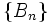$\{ B_n \}$

To prove: If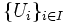$\{ U_i \}_{i \in I}$ form an open cover of$X$, there exists a countable subcover of$X$ among the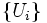$\{ U_i \}$s

Proof: For each basis element$B_n$, let$V_n$ be any$U_i$ containing$B_n$, if such a$U_i$ exists, otherwise, pick nothing. This gives a (at most) countable subcollection$\{ V_n \}$ of the collection$\{ U_i \}$. We want to show that this subcollection covers$X$.

Suppose there exists$x \in X$, that does not belong to any$V_n$. Then, since the entire collection of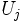$U_j$ cover$X$, we can find some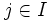$j \in I$ such that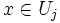$x \in U_j$. Further, there exists some$n$ such that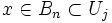$x \in B_n \subset U_j$. Now, since$B_n$ is contained in at least one of the$U_i$s, there should exist an element$V_n$. But such an element would also contain$x$, contradicting the claim that$x$ does not belong to any$V_n$.